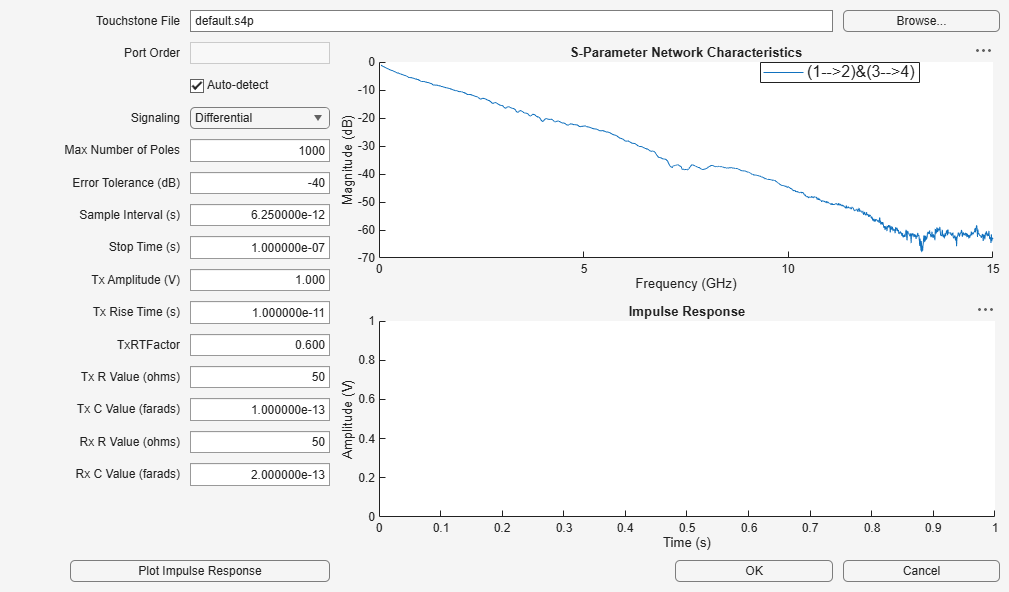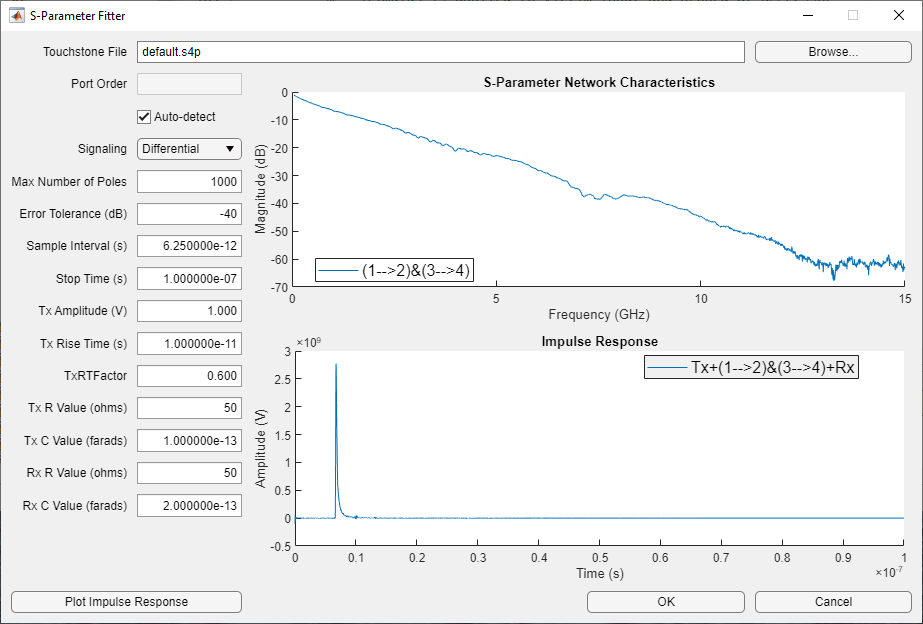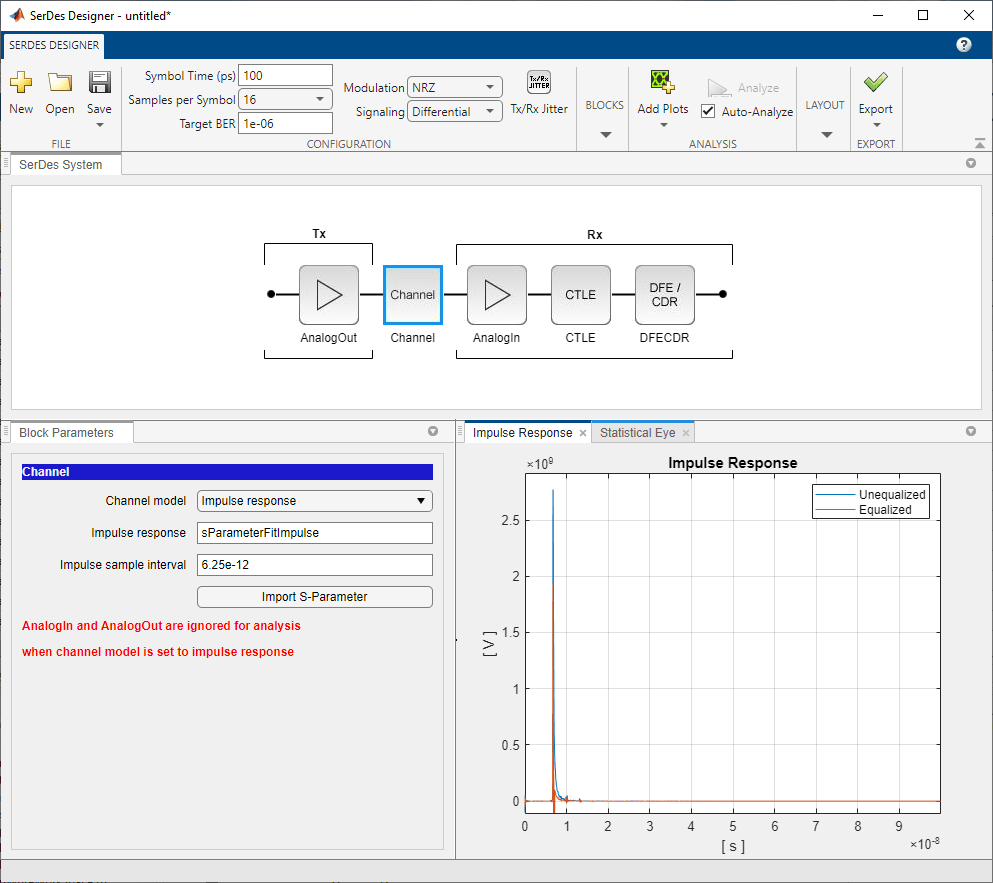# Convert Scattering Parameter to Impulse Response for SerDes System

This example covers two topics: the first shows how to combine a Scattering-Parameter (S-Parameter) model of a baseband communication channel with an analog transmitter and receiver into a single 4-port S-Parameter. The second shows a possible method to fit a transfer function to the S-Parameter and find its impulse response for use with SerDes Toolbox™ using the functions `rational` (RF Toolbox) and `impulse` (RF Toolbox) from RF Toolbox™.

### Configure Variables

The S-Parameter file representing the baseband channel should be a 4-port .`s4p` file with a reference impedance of 50-Ohms (100-Ohms differential). For different channel impedance (such as 42.5-Ohms single-ended or 85-Ohms differential), you may reconfigure the analog transmitter and receiver values to match. Also, you will see in this example the values for `Datarate` is set to 10 GHz and `SamplesPerSymb` is set to 32, and these values may be different for your system. You can adjust the rise time and amplitude of the transmitter using `TxRiseTime` and `TxAmplitude`, respectively, and adjust the duration of the calculated impulse response using `endTime`. Also, you can optimize the fit model using the function `rational` by adjusting the control parameters `errorTolerance` and `nPoles`.

```showPlots = true; %Define system data rate and discrete sampling dataRate = 10*1e9; samplesPerSymbol = 32; stopTime = 100e-9; %(seconds) set the time duration of impulse response %Specify channel S-parameter ChannelFilename = 'default.s4p'; %Define transmitter analog model TxR = 50; % Ohms TxC = 0.1e-12; %Farads TxAmplitude = 1.2; %Voltage TxRiseTime2080 = 40e-12; % 20/80% rise time %Define receiver analog model RxR = 50; %Ohms RxC = 0.2e-12; %Farads %Specify rational fitting parameters nPoles = 1600; %Maximum number of poles to use errTolerance = -50; %(dB) desired error tolerance of fit %% Form channel modeling including transmitter/receiver termination Rshort = 1.0e-6;```

### Import Differential S-Parameter Model of Communication Channel

Import the S-Parameter data file and keep the original frequency content.

```%% Import Differential S-Parameter Model of Communication Channel %Import the S-Parameter data file and keep the original frequency content. origSparam = sparameters(ChannelFilename); freq = origSparam.Frequencies;```

### Combine S-Parameter of Communication Channel with Analog Sections of Transmitter and Receiver

You can see how to use MATLAB to convert impedance characteristics of the analog Tx and Rx circuits into S-Parameter equivalents. These can then be combined with the S-Parameter of the Communication Channel to create an S-Parameter model of the system.

#### Convert Analog Model of Transmitter to S-Parameter

```cktTx = circuit('txAnalog'); add(cktTx,[1 3],resistor(TxR,'R1')) add(cktTx,[3 0],capacitor(TxC,'C1')) add(cktTx,[2 4],resistor(TxR,'R2')) add(cktTx,[4 0],capacitor(TxC,'C2')) setports(cktTx,[1 0],[2 0],[3 0],[4 0]) StxAnalog = sparameters(cktTx,freq,50);```

#### Configure Port Order for S-Parameter of Communication Channel

```cktCh = circuit('ChannelSparam'); channel = nport(ChannelFilename); % Create s-parameter circuit element from the 4-port S-parameter file. add(cktCh,[1 3 2 4],channel); setports(cktCh,[1 0],[2 0],[3 0],[4 0]) Schannel = sparameters(cktCh,freq,50);```

#### Convert Analog Model of Receiver to S-Parameter

```cktRx = circuit('rxAnalog'); add(cktRx,[1 0],resistor(RxR,'R3')) add(cktRx,[1 0],capacitor(RxC,'C3')) add(cktRx,[2 0],resistor(RxR,'R4')) add(cktRx,[2 0],capacitor(RxC,'C4')) add(cktRx,[1 3],resistor(Rshort,'R5')) add(cktRx,[2 4],resistor(Rshort,'R6')) setports(cktRx,[1 0],[2 0],[3 0],[4 0]) SrxAnalog = sparameters(cktRx,freq,50);```

### Create Combined S-Parameter Object

You can combine the S-Parameters using `cascadesparams`. Set values of `data`, `freq` and `z0` for use by the `rational` function. Use `s2sdd` to convert the data to a 4-port differential S-Parameter `diffdata`. Note: RF Toolbox™ applies ports first from top to bottom on an S-parameter, then from left to right. Be aware that ports on an imported S-Paramter may be formatted as left-to-right, then top-to-bottom, and may have to be re-configured for use by RF Toolbox™.

```sparamWithAnalog = cascadesparams(StxAnalog,Schannel,SrxAnalog); data = sparamWithAnalog.Parameters; freq = sparamWithAnalog.Frequencies; z0 = sparamWithAnalog.Impedance; diffdata = s2sdd(data,2); diffz0 = 2*z0; diffsparams = sparameters(diffdata,freq,diffz0);```

### Convert S-Parameter to Transfer Function

You can find the transfer function using the MATLAB function `s2tf` using the control parameters for reference impedance `zRef,` source impedance `zSource` and load impedance `zLoad`.

Note: The source impedance is set to short-circut and load impedance is set to open-circuit, because the impedance characteristics of the Tx and Rx are already included S-Parameter model of the LTI system.

```zRef = diffz0; % Reference impedance of S-Parameters zSource = 1e-6; % Short circuit the source impedance since it is included in the Tx Analog model. zLoad = 1e6; % Open circuit the load impedance since it is included in the Rx Analog model. difftransfunc = s2tf(diffdata,zRef,zSource,zLoad);```

### Find Fit for theTransfer Function by using Rational Function

You can see how to use rational to obtain a fit:

Note: for the function `rational`, it is recommended to set TendsToZero to `true` for fast TX rise times in order to mitigate potential numerical artifacts in the impulse response.

```%Fit model to transfer function rationalResults = rational(freq,difftransfunc,errTolerance,'TendsToZero',true,'MaxPoles',nPoles,'Display',"off"); fprintf('\nThe derived rational function achieved %f dB fit with %d poles.\n',rationalResults.ErrDB,rationalResults.NumPoles);```
```The derived rational function achieved -51.339448 dB fit with 160 poles. ```
```%% Extract impulse response from rational fit results %Determine symbol time, time steps and duration (in samples) of the impulse SymbolTime = 1/dataRate; ts = SymbolTime/samplesPerSymbol; pointsInImpulse = round(stopTime/ts);```

### Find the Impulse Response using Impulse and Rational Output

Use the function `impulse` to find the impulse response of the fit:

`impulseRaw = impulse(rationalResults,ts,pointsInImpulse);`

### Modelling TX Rise Time and Amplitude

You can include TX rise time and amplitude by convolving a rectangular pulse with the impulse response. Note: You can convert a 20-80% to 0-100% rise time assuming a linear ramp.

```TxRiseTime = TxRiseTime2080/0.6; risetimeSamples = round(TxRiseTime/ts); %Determine width of rise time pulse```

### Using a Linear Ramp Model for Rise Time

Note: Using a linear ramp model of the rise time in a discrete time system leads to the possibility of some uncertainty of using a linear ramp with given sampling interval. A reduction in uncertainty can be had by increasing the sampling resolution by increasing samples per symbol.

```riseTimeUncertainty = (TxRiseTime - risetimeSamples*ts)/TxRiseTime*100; fprintf('Rise time uncertainty is %g %%\n',riseTimeUncertainty);```
```Rise time uncertainty is 1.5625 % ```
```%Create rise time pulse response riseTimePulse = zeros(risetimeSamples*2,1); riseTimePulse(1:risetimeSamples) = 1/risetimeSamples; %Convolve rise time pulse with raw impulse response and scale by tx amplitude. if risetimeSamples>1 impulseResponse = TxAmplitude*conv(impulseRaw,riseTimePulse,'same'); else impulseResponse = TxAmplitude*impulseRaw; end t = (0:length(impulseResponse)-1)*ts;```

### Compare Fit Results to Original Data

You can compare the original data and fit differential-mode frequency response by plotting the magnitude and phase of the original transfer function and the esulting output of the `rational` function.

```%Extract frequency response from fit freqsforresp = linspace(0,max(freq)*2,length(freq))'; resp = freqresp(rationalResults,freqsforresp); figure(1001) subplot(2,1,1) plot(freq*1.e-9,20*log10(abs(difftransfunc)),'r',... freqsforresp*1.e-9, 20*log10(abs(resp)),'b--','LineWidth',2) title(sprintf('Rational Fitting with %d poles',rationalResults.NumPoles),'FontSize',12) ylabel('Magnitude (decibels)') xlabel('Frequency (GHz)') legend('Original data','Fit result') grid on subplot(2,1,2) origangle = unwrap(angle(difftransfunc))*180/pi; plotangle = unwrap(angle(resp))*180/pi; plot(freq*1.e-9,origangle,'r',freqsforresp*1.e-9,plotangle,'b--', ... 'LineWidth',2) ylabel('Phase (deg.)') xlabel('Frequency (GHz)') legend('Original data','Fit result') grid on```### Plot Impulse Response

Here you can see a plot of the Impulse Response for the combination of the analog portion of the transmitter, baseband channel, and analog portion of the receiver.

```%% Visualize impulse response figure(1002) plot(t*1e9,impulseResponse) xlabel('Time (ns)') ylabel('Volts') title(sprintf('Impulse Response derived from\n%s\nand Tx/Rx analog model',ChannelFilename),'interpreter','none') grid on```### Use Impulse Response for Channel Model in Serdes Designer

You can use the impulse response of the baseband channel model within Serdes Designer by selecting "Impulse response" for channel model and enter the vector `impulseResponse`.